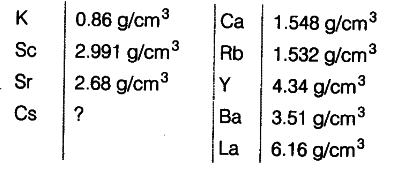# Predict the density of Cs from the density of the following elements

Predict the density of Cs from the density of the following elements.Density of Cs = 2.68+1.532 / 2 = 4.212 / 2 = 2.106 g / \${{cm}^{3}}\$